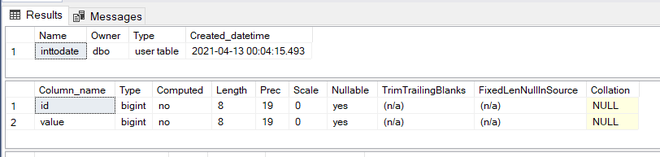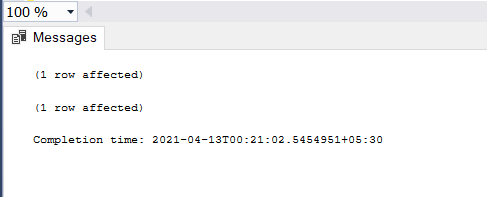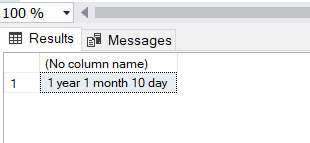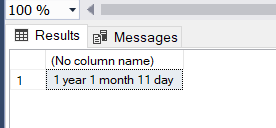# SQL Query to Convert an Integer to Year Month and Days

• Difficulty Level : Expert
• Last Updated : 26 Apr, 2021

With this article, we will be knowing how to convert an integer to Year, Month, Days from an integer value. The prerequisites of this article are you should be having a MSSQL server on your computer.

#### What is a query?

A query is a statement or a group of statements written to perform a specific task, like retrieve data, save data into a database.

Let us make a database first to perform this task –

#### Step 1:

`CREATE DATABASE GFG`

Step 2: Using the database

`USE GFG`

Step 3: Create a table with name inttodate –

```CREATE TABLE inttodate (id bigint,
value bigint);```

Step 4: Describe this table –Created Table schema

Step 5: Insert some values to the table –

```INSERT INTO [dbo].[inttodate]
([id]
,[value])
VALUES
(1
,35110011)

INSERT INTO [dbo].[inttodate]
([id]
,[value])
VALUES
(2
,35220011)```Data inserted into the table

Step 6: Now run the query to check what is the year, month, day conversion of the inserted data –

Here we will be creating a variable using dateadd function, and then we will split the values into the year, month and date.

```DECLARE @variable_date DATETIME = DATEADD(SECOND, (SELECT [value]
FROM [dbo].[inttodate] WHERE [id] = '1'), 0)

SELECT CAST(DATEPART(YEAR, @variable_date) - 1900 AS VARCHAR(10))
+ ' year ' + CAST(DATEPART(MONTH, @variable_date) - 1 AS VARCHAR(2)) + ' month '
+ CAST(DATEPART(DD, @variable_date) - 1 AS VARCHAR(2)) + ' day '```Output when id =1 is used

Step 7: Check with different data when id = 2 –

```DECLARE @variable_date DATETIME = DATEADD(SECOND, (SELECT [value]
FROM [dbo].[inttodate] WHERE [id] = '2'), 0)

SELECT CAST(DATEPART(YEAR, @variable_date) - 1900 AS VARCHAR(10)) + ' year '
+ CAST(DATEPART(MONTH, @variable_date) - 1 AS VARCHAR(2)) + ' month '
+ CAST(DATEPART(DD, @variable_date) - 1 AS VARCHAR(2)) + ' day '```Output when id = 2 is used

So we are getting desired output.

My Personal Notes arrow_drop_up